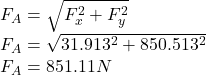## he uniform 110-kg beam is freely hinged about its upper end A and is initially at rest in the vertical position withθ= 0. Determine the init

Question

he uniform 110-kg beam is freely hinged about its upper end A and is initially at rest in the vertical position withθ= 0. Determine the initial angular accelerationα of the beam and the magnitudeFAof the force supported by the pin at A due to the application of the force P = 350 N on the attached cab

in progress 0
2 months 2021-07-31T07:08:39+00:00 2 Answers 1 views 0

The initial angular acceleration of the beam is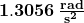The magnitude of the force at A is 832.56N

Explanation:

Here, m is the mass of the beam and l is the length of the beam.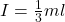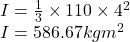Take the moment about point A by applying moment equilibrium equation.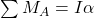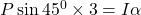Here, P is the force applied to the attached cable and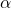is the angular acceleration.

Substitute 350 for P and 586.67kg.m² for I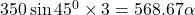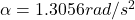The initial angular acceleration of the beam isFind the acceleration along x direction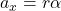Here, r is the distance from center of mass of the beam to the pin joint A.

Substitute 2 m for r and 1.3056rad/s² for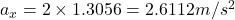Find the acceleration along the y direction.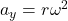Here, ω is angular velocity.

Since beam is initially at rest,ω=0

Substitute 0 for ω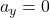Apply force equilibrium equation along the horizontal direction.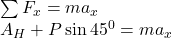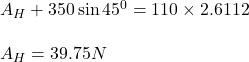Apply force equilibrium equation along the vertical direction.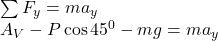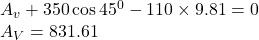Calculate the resultant force,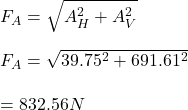The magnitude of the force at A is 832.56N

a) Initial angular acceleration of the beam = 1.27 rad/s²

b)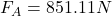Explanation: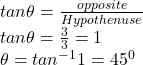Force applied to the attached cable, P = 350 N

Mass of the beam, m = 110-kg

Mass moment of the inertia of the beam about point A =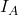Using the parallel axis theorem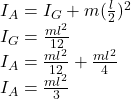Moment = Force * Perpendicular distance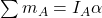From the free body diagram drawn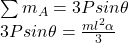P = 350 N, l = 3+ 1 = 4 m, θ = 45°

Substitute these values into the equation above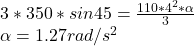Linear acceleration along the x direction is given by the formula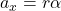r = 2 m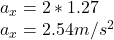the linear acceleration along the y-direction is given by the formula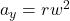Since the beam is initially at rest, w = 0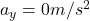General equation of motion along x – direction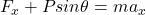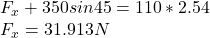General equation of motion along y – direction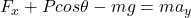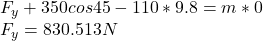Magnitude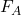of the force supported by the pin at A